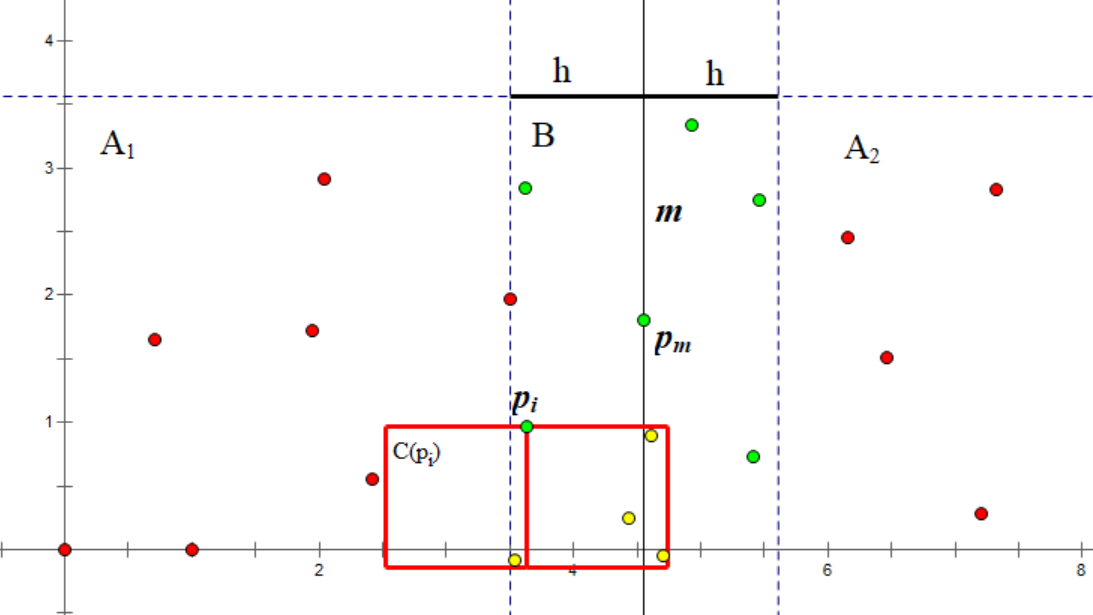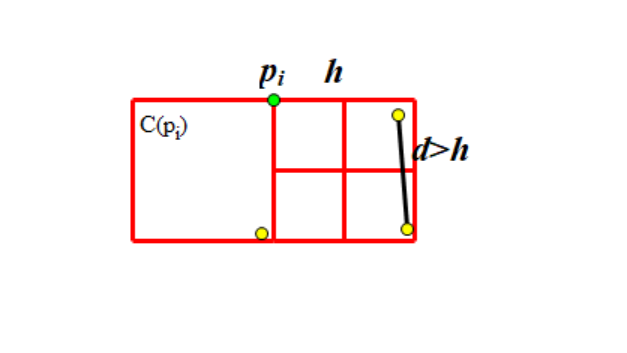# 平面最近点对

## 算法¶1. 构建集合
2. 中的点按照 排序。通常做法是 ，但是我们可以改变策略优化到 （下文讲解）。
3. 对于每个 考虑 ，对于每对 计算距离并更新答案（当前所处集合的最近点对）。

## 复杂度证明¶## 实现¶

  1 2 3 4 5 6 7 8 9 10 11 12 13 14 15 16 struct pt { int x, y, id; }; struct cmp_x { bool operator()(const pt& a, const pt& b) const { return a.x < b.x || (a.x == b.x && a.y < b.y); } }; struct cmp_y { bool operator()(const pt& a, const pt& b) const { return a.y < b.y; } }; int n; vector a; 

 1 2 3 4 5 6 7 8 double mindist; int ansa, ansb; inline void upd_ans(const pt& a, const pt& b) { double dist = sqrt((a.x - b.x) * (a.x - b.x) + (a.y - b.y) * (a.y - b.y) + .0); if (dist < mindist) mindist = dist, ansa = a.id, ansb = b.id; } 

  1 2 3 4 5 6 7 8 9 10 11 12 13 14 15 16 17 18 19 20 21 22 void rec(int l, int r) { if (r - l <= 3) { for (int i = l; i <= r; ++i) for (int j = i + 1; j <= r; ++j) upd_ans(a[i], a[j]); sort(a + l, a + r + 1, &cmp_y); return; } int m = (l + r) >> 1; int midx = a[m].x; rec(l, m), rec(m + 1, r); inplace_merge(a + l, a + m + 1, a + r + 1, &cmp_y); static pt t[MAXN]; int tsz = 0; for (int i = l; i <= r; ++i) if (abs(a[i].x - midx) < mindist) { for (int j = tsz - 1; j >= 0 && a[i].y - t[j].y < mindist; --j) upd_ans(a[i], t[j]); t[tsz++] = a[i]; } } 

 1 2 3 sort(a, a + n, &cmp_x); mindist = 1E20; rec(0, n - 1); 

## 非分治算法¶

1. 将所有满足 的点从集合中删除。它们不会再对答案有贡献。
2. 对于集合内满足 的所有点，统计它们和 的距离。
3. 插入到集合中。

  1 2 3 4 5 6 7 8 9 10 11 12 13 14 15 16 17 18 19 20 21 22 23 24 25 26 27 28 29 30 31 32 33 34 35 36 37 38 39 40 41 42 43 44 45 46 #include #include #include #include const int N = 200005; int n; double ans = 1e20; struct point { double x, y; point(double x = 0, double y = 0) : x(x), y(y) {} }; struct cmp_x { bool operator()(const point &a, const point &b) const { return a.x < b.x || (a.x == b.x && a.y < b.y); } }; struct cmp_y { bool operator()(const point &a, const point &b) const { return a.y < b.y; } }; inline void upd_ans(const point &a, const point &b) { double dist = sqrt(pow((a.x - b.x), 2) + pow((a.y - b.y), 2)); if (ans > dist) ans = dist; } point a[N]; std::multiset s; int main() { scanf("%d", &n); for (int i = 0; i < n; i++) scanf("%lf%lf", &a[i].x, &a[i].y); std::sort(a, a + n, cmp_x()); for (int i = 0, l = 0; i < n; i++) { while (l < i && a[i].x - a[l].x >= ans) s.erase(s.find(a[l++])); for (auto it = s.lower_bound(point(a[i].x, a[i].y - ans)); it != s.end() && it->y - a[i].y < ans; it++) upd_ans(*it, a[i]); s.insert(a[i]); } printf("%.4lf", ans); return 0; }